## AVERAGEIF Function

The AVERAGEIF function is a premade function in Google Sheets, which calculates the average of a range based on a true or false condition.

It is typed `=AVERAGEIF` and has three parts:

=AVERAGEIF(criteria_range, criterion, [average_range])

The condition is referred to as `criterion`, which can check things like:

• If a number is greater than another number `>`
• If a number is smaller than another number `<`
• If a number or text is equal to something `=`

The `[average_range]` is the range where the function calculates the average.

Note: The `[average_range]` is optional.

If not specified, the function calculates the average of the same range as condition.

## Example AVERAGEIF function

Find the average speed of Grass type Pokemon:

The condition is that the type is "Grass".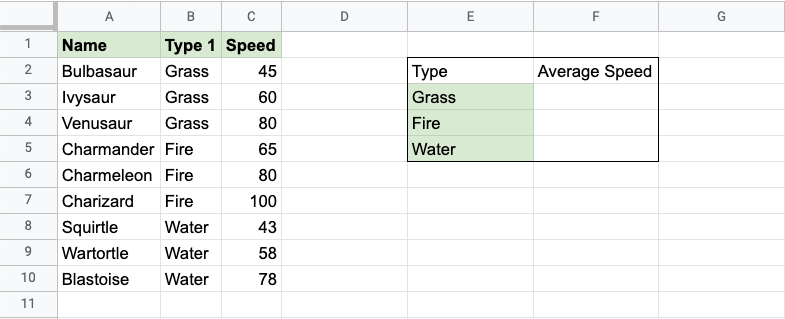Copy Values

Example AVERAGEIF function, step by step:

1. Select the cell `F3`
2. Type `=AVERAGEIF`
3. Click the AVERAGEIF command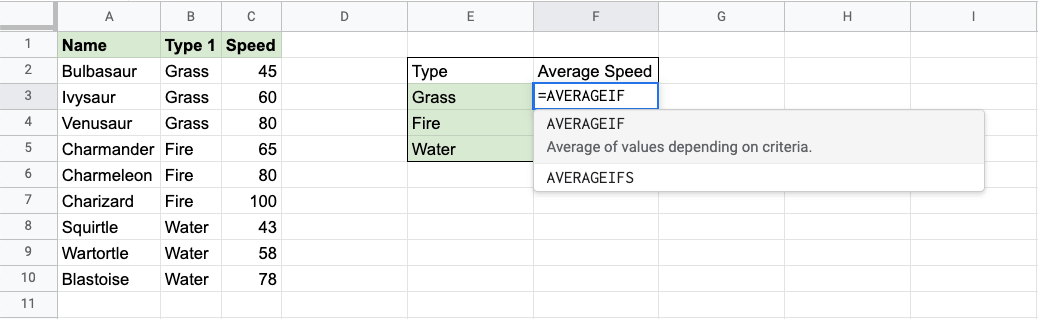1. Specify the range for the condition `B2:B10` (the Type 1 values)
2. Type `,`
3. Specify the criteria (the cell `E3`, which has the value "Grass")
4. Type `,`
5. Specify the range for the average `C2:C10` (the Speed values)
6. Hit enter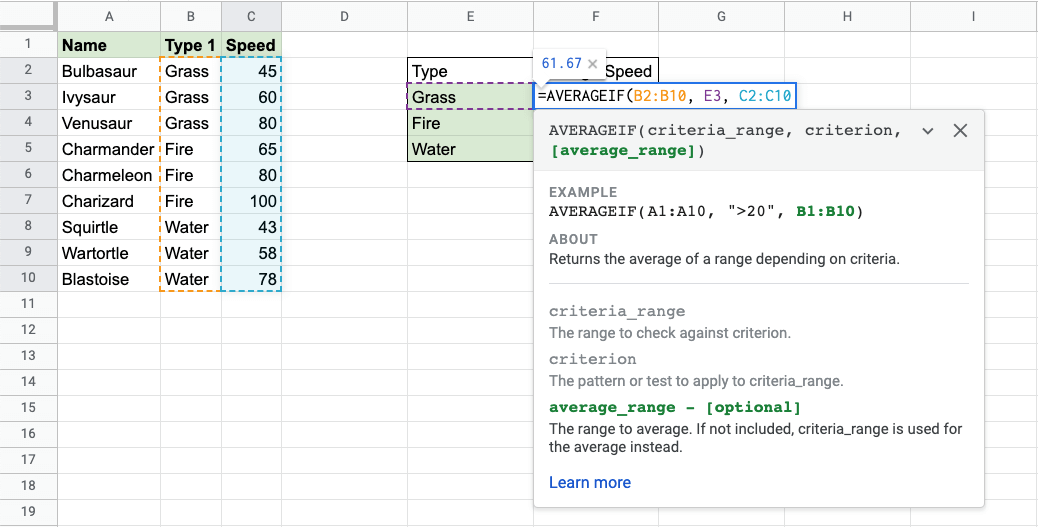The function now calculates the average speed value of the Grass type Pokemon: Bulbasaur, Ivysaur and Venusaur.

The function can be repeated for Fire and Water type Pokemon to compare them: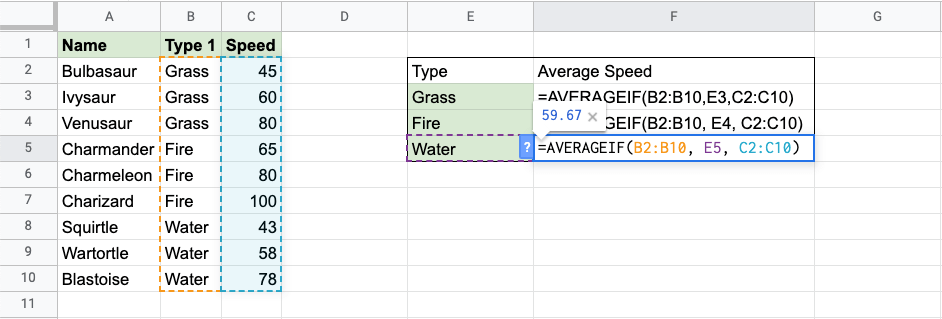Note: You can use the filling function for the other rows, but make sure to use absolute references for the ranges.

Now, we can see the average speed values of each type: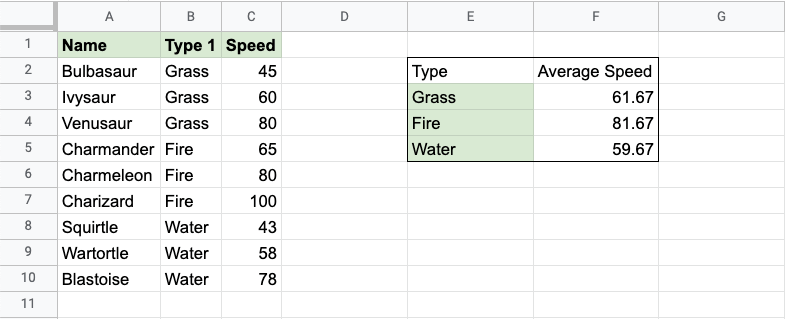W3Schools is optimized for learning and training. Examples might be simplified to improve reading and learning. Tutorials, references, and examples are constantly reviewed to avoid errors, but we cannot warrant full correctness of all content. While using W3Schools, you agree to have read and accepted our terms of use, cookie and privacy policy.